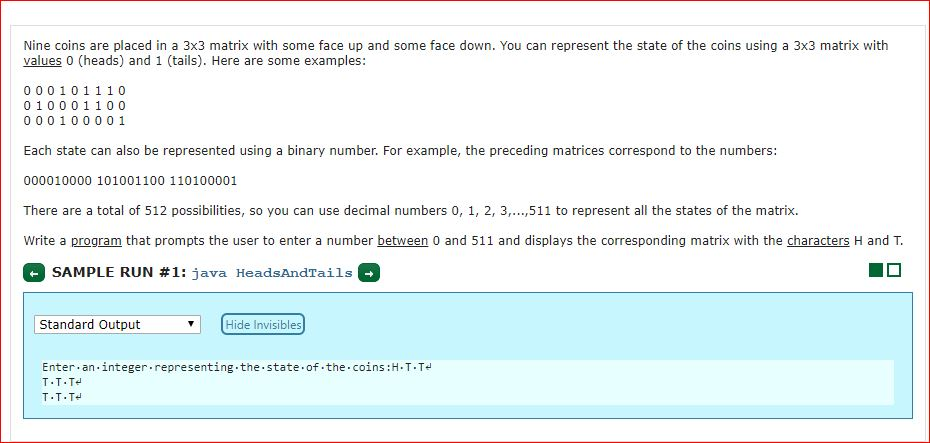# Nine coins are placed in a 3x3 matrix with some face up and some face down. You can represent the state of the coins using a 3x3 matrix withvalues 0 (heads) and 1 (tails). Here are some examples:00 0 1 0 1 1 100 10 0 0 1 10000010000 1Each state can also be represented using a binary number. For example, the preceding matrices correspond to the numbers:000010000 101001100 110100001There are a total of 512 possibilities, so you can use decimal numbers 0, 1, 2, 3.,511 to represent all the states of the matrix.Write a program that prompts the user to enter a number between 0 and 511 and displays the corresponding matrix with the characters H and T.SAMPLE RUN #1: java HeadsAndTailsStandard OutputHide InvisiblesEnter.an integer representing.the.state.of.the.coins:H.T.TT T.T

Question
546 views

Nine coins are placed in a 3x3 matrix with some face up and some face down. You can represent the state of the coins using a 3x3 matrix with values 0 (heads) and 1 (tails). Here are some examples:

0 0 0 1 0 1 1 1 0
0 1 0 0 0 1 1 0 0
0 0 0 1 0 0 0 0 1

Each state can also be represented using a binary number. For example, the preceding matrices correspond to the numbers:

000010000 101001100 110100001

There are a total of 512 possibilities, so you can use decimal numbers 0, 1, 2, 3,...,511 to represent all the states of the matrix.

Write a program that prompts the user to enter a number between 0 and 511 and displays the corresponding matrix with the characters H and T.help_outlineImage TranscriptioncloseNine coins are placed in a 3x3 matrix with some face up and some face down. You can represent the state of the coins using a 3x3 matrix with values 0 (heads) and 1 (tails). Here are some examples: 00 0 1 0 1 1 10 0 10 0 0 1 100 00010000 1 Each state can also be represented using a binary number. For example, the preceding matrices correspond to the numbers: 000010000 101001100 110100001 There are a total of 512 possibilities, so you can use decimal numbers 0, 1, 2, 3.,511 to represent all the states of the matrix. Write a program that prompts the user to enter a number between 0 and 511 and displays the corresponding matrix with the characters H and T. SAMPLE RUN #1: java HeadsAndTails Standard Output Hide Invisibles Enter.an integer representing.the.state.of.the.coins:H.T.T T T.T fullscreen
check_circle

Step 1

As there is no programming language mentioned, we are writing this program in JAVA. The program is given below:

Step 2

import java.util.Scanner;

{

public static void main(String[] args)

{

Scanner ht = new Scanner(System.in);

System.out.print("Enter number between 0 and 511: ");

int input = ht.nextInt();

String binary = Integer.toBinaryString(input); // Turning the input to binary string

binary = binary.format("%09d", Integer.parseInt(binary)); // Adding zeros in front so that the string has 9 characters

for (int i = 1; i < 10; i++) // Iterate one char at a time through binary string

{

if ('0' == binary.charAt(i - 1))

{

System.out.print("H ");

}

else

{

System.out.print("T ");

}

if (i % 3 == 0) // New line after 3 letters

{

System.out.println();

}

}

}

}

Step 3

In the above java program, a class is created with the name Headtail and the number is inputted through the user. Then the given input is converted into the binary string using the function Integer.toBinaryStrin...

### Want to see the full answer?

See Solution

#### Want to see this answer and more?

Solutions are written by subject experts who are available 24/7. Questions are typically answered within 1 hour.*

See Solution
*Response times may vary by subject and question.
Tagged in

### Computer Science